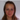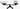Product
Company
Social

# Best practices of orchestrating Python and R code in ML projects

Today, data scientists are generally divided among two languages — some prefer R, some prefer Python. I will try to find an answer to a question: “What is the best way to integrate both languages in one data science project? What are the best practices?”• Marija Ilić
• September 26, 201710 min. readImage was taken from intersog.com

Beside Git and shell scripting additional tools are developed to facilitate the development of predictive model in a multi-language environments. For fast data exchange between R and Python let’s use binary data file format Feather. Another language agnostic tool DVC can make the research reproducible — let’s use DVC to orchestrate R and Python code instead of a regular shell scripts.

## Machine learning with R and Python

Both R and Python are having powerful libraries/packages used for predictive modeling. Usually algorithms used for classification or regression are implemented in both languages and some scientist are using R while some of them preferring Python. In an example that was explained in previous tutorial target variable was binary output and logistic regression was used as a training algorithm. One of the algorithms that could also be used for prediction is a popular Random Forest algorithm which is implemented in both programming languages. Because of performances it was decided that Random Forest classifier should be implemented in Python (it shows better performances than random forest package in R).

## R example used for DVC demo

We will use the same example from previous blog story, add some Python codes and explain how Feather and DVC can simplify the development process in this combined environment.

Let’s recall briefly the R codes from previous tutorial:

Input data are StackOverflow posts — an XML file. Predictive variables are created from text posts — relative importance tf-idf of words among all available posts is calculated. With tf-idf matrices target is predicted and lasso logistic regression for predicting binary output is used. AUC is calculated on the test set and AUC metric is used on evaluation.

Instead of using logistic regression in R we will write Python jobs in which we will try to use random forest as training model. Train_model.R and evaluate.R will be replaced with appropriate Python jobs.

R codes can be seen here.

Code for `train_model_Python.py` is presented below:

 import numpy as np from sklearn.ensemble import RandomForestClassifier import sys try: import cPickle as pickle # python2 except: import pickle # python3 from scipy import sparse from numpy import loadtxt import feather as ft if len(sys.argv) != 4: sys.stderr.write('Arguments error. Usage:\n') sys.stderr.write('\tpython train_model.py INPUT_MATRIX_FILE SEED OUTPUT_MODEL_FILE\n') sys.exit(1) input = sys.argv seed = int(sys.argv) output = sys.argv df = ft.read_dataframe(input) labels = df.loc[:,'label'] x = df.loc[:, df.columns != 'label'] clf = RandomForestClassifier(n_estimators=100, n_jobs=2, random_state=seed) clf.fit(x, labels.ix[:,0]) with open(output, 'wb') as fd: pickle.dump(clf, fd)

Also here we are adding code for `evaluation_python_model.py`:

 from sklearn.metrics import precision_recall_curve import sys import sklearn.metrics as metrics from scipy import sparse from numpy import loadtxt try: import cPickle as pickle # python2 except: import pickle # python3 import feather as ft if len(sys.argv) != 4: sys.stderr.write('Arguments error. Usage:\n') sys.stderr.write('\tpython metrics.py MODEL_FILE TEST_MATRIX METRICS_FILE\n') sys.exit(1) model_file = sys.argv test_matrix_file = sys.argv metrics_file = sys.argv with open(model_file, 'rb') as fd: model = pickle.load(fd) df = ft.read_dataframe(test_matrix_file) labels = df.loc[:,'label'] x = df.loc[:, df.columns != 'label'] predictions_by_class = model.predict_proba(x) predictions = predictions_by_class[:,1] precision, recall, thresholds = precision_recall_curve(labels.ix[:,0], predictions) auc = metrics.auc(recall, precision) #print('AUC={}'.format(metrics.auc(recall, precision))) with open(metrics_file, 'w') as fd: fd.write('AUC: {:4f}\n'.format(auc))

Let’s download necessary R and Python codes from above (clone the Github repository):

``````\$ mkdir R_DVC_GITHUB_CODE
\$ cd R_DVC_GITHUB_CODE

\$ git clone https://github.com/Zoldin/R_AND_DVC``````

Our dependency graph of this data science project look like this:

R (marked red) and Python (marked pink) jobs in one project

Now lets see how it is possible to speed up and simplify process flow with Feather API and data version control reproducibility.

## Feather API

Feather API is designed to improve meta data and data interchange between R and Python. It provides fast import/export of data frames among both environments and keeps meta data information which is an improvement over data exchange via csv/txt file format. In our example Python job will read an input binary file that was produced in R with Feather api.

Let’s install Feather library in both environments.

For Python 3 on linux environment you can use cmd and pip3:

``\$ sudo pip3 install feather-format``

For R it is necessary to install feather package:

``install.packages(feather)``

After successful installation we can use Feather for data exchange.

Below is an R syntax for data frame export with Feather (featurization.R):

``````library(feather)

write_feather(dtm_train_tfidf,args)
write_feather(dtm_test_tfidf,args)
print("Two data frame were created with Feather - one for train and one for test data set")``````

Python syntax for reading feather input binary files (trainmodelpython.py):

``````import feather as ft

input = sys.argv

## Dependency graph with R and Python combined

The next question what we are asking ourselves is why do we need DVC, why not just use shell scripting? DVC automatically derives the dependencies between the steps and builds the dependency graph (DAG) transparently to the user. Graph is used for reproducing parts/codes of your pipeline which were affected by recent changes and we don’t have to think all the time what we need to repeat (which steps) with the latest changes.

Firstly, with `dvc run` command we will execute all jobs that are related to our model development. In that phase DVC creates dependencies that will be used in the reproducibility phase:

``````\$ dvc import https://s3-us-west-2.amazonaws.com/dvc-share/so/25K/Posts.xml.tgz \
data/

\$ dvc run tar zxf data/Posts.xml.tgz -C data/

\$ dvc run Rscript code/parsingxml.R \
data/Posts.xml data/Posts.csv

\$ dvc run Rscript code/train_test_spliting.R \
data/Posts.csv 0.33 20170426 \
data/train_post.csv data/test_post.csv

\$ dvc run Rscript code/featurization.R \
data/train_post.csv \
data/test_post.csv data/matrix_train.feather \
data/matrix_test.feather

\$ dvc run python3 code/train_model_python.py \
data/matrix_train.feather \
20170426 data/model.p

\$ dvc run python3 code/evaluate_python_mdl.py \
data/model.p data/matrix_test.feather \
data/evaluation_python.txt``````

After this commands jobs are executed and included in DAG graph. Result (AUC metrics) is written in evaluation_python.txt file:

``````\$ cat data/evaluation_python.txt
AUC: 0.741432``````

It is possible to improve our result with random forest algorithm.

We can increase number of trees in the random forest classifier — from 100 to 500:

``````clf = RandomForestClassifier(n_estimators=500,
n_jobs=2,
random_state=seed)
clf.fit(x, labels)``````

After commited changes (in `train_model_python.py`) with `dvc repro` command all necessary jobs for `evaluation_python.txt` reproduction will be re-executed. We don’t need to worry which jobs to run and in which order.

``````\$ git add .
\$ git commit
[master a65f346] Random forest classifier — more trees added
1 file changed, 1 insertion(+), 1 deletion(-)

\$ dvc repro data/evaluation_python.txt

Reproducing run command for data item data/model.p. Args: python3 code/train_model_python.py data/matrix_train.txt 20170426 data/model.p
Reproducing run command for data item data/evaluation_python.txt. Args: python3 code/evaluate_python_mdl.py data/model.p data/matrix_test.txt data/evaluation_python.txt
Data item “data/evaluation_python.txt” was reproduced.``````

Beside code versioning, DVC also cares about data versioning. For example, if we change data sets `train_post.csv` and `test_post.csv` (use different splitting ratio) DVC will know that data sets are changed and `dvc repro` will re-execute all necessary jobs for evaluation_python.txt.

``````\$ dvc run Rscript code/train_test_spliting.R \
data/Posts.csv 0.15 20170426 \
data/train_post.csv \
data/test_post.csv``````

Re-executed jobs are marked with red color:

``````\$ dvc run Rscript code/train_test_spliting.R \
data/Posts.csv 0.15 20170426 \
data/train_post.csv \
data/test_post.csv

\$ dvc repro data/evaluation_python.txt

Reproducing run command for data item data/matrix_train.txt. Args: Rscript — vanilla code/featurization.R data/train_post.csv data/test_post.csv data/matrix_train.txt data/matrix_test.txt
Reproducing run command for data item data/model.p. Args: python3 code/train_model_python.py data/matrix_train.txt 20170426 data/model.p
Reproducing run command for data item data/evaluation_python.txt. Args: python3 code/evaluate_python_mdl.py data/model.p data/matrix_test.txt data/evaluation_python.txt

Data item “data/evaluation_python.txt” was reproduced.

\$ cat data/evaluation_python.txt
AUC: 0.793145``````

New AUC result is 0.793145 which shows an improvement compared to previous iteration.

## Summary

In data science projects it is often used R/Python combined programming. Additional tools beside git and shell scripting are developed to facilitate the development of predictive model in a multi-language environments. Using data version control system for reproducibility and Feather for data interoperability helps you orchestrate R and Python code in a single environment.

R
Python
DVC
Tutorial
Best PracticesSubscribe for updates. We won't spam you.Product
Company
Social